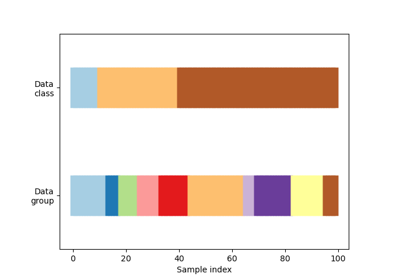# sklearn.model_selection.GroupKFold¶

class sklearn.model_selection.GroupKFold(n_splits=5)[source]

K-fold iterator variant with non-overlapping groups.

The same group will not appear in two different folds (the number of distinct groups has to be at least equal to the number of folds).

The folds are approximately balanced in the sense that the number of distinct groups is approximately the same in each fold.

Read more in the User Guide.

Parameters
n_splitsint, default=5

Number of folds. Must be at least 2.

Changed in version 0.22: n_splits default value changed from 3 to 5.

LeaveOneGroupOut

For splitting the data according to explicit domain-specific stratification of the dataset.

Examples

>>> import numpy as np
>>> from sklearn.model_selection import GroupKFold
>>> X = np.array([[1, 2], [3, 4], [5, 6], [7, 8]])
>>> y = np.array([1, 2, 3, 4])
>>> groups = np.array([0, 0, 2, 2])
>>> group_kfold = GroupKFold(n_splits=2)
>>> group_kfold.get_n_splits(X, y, groups)
2
>>> print(group_kfold)
GroupKFold(n_splits=2)
>>> for train_index, test_index in group_kfold.split(X, y, groups):
...     print("TRAIN:", train_index, "TEST:", test_index)
...     X_train, X_test = X[train_index], X[test_index]
...     y_train, y_test = y[train_index], y[test_index]
...     print(X_train, X_test, y_train, y_test)
...
TRAIN: [0 1] TEST: [2 3]
[[1 2]
[3 4]] [[5 6]
[7 8]] [1 2] [3 4]
TRAIN: [2 3] TEST: [0 1]
[[5 6]
[7 8]] [[1 2]
[3 4]] [3 4] [1 2]


Methods

 get_n_splits([X, y, groups]) Returns the number of splitting iterations in the cross-validator split(X[, y, groups]) Generate indices to split data into training and test set.
get_n_splits(X=None, y=None, groups=None)[source]

Returns the number of splitting iterations in the cross-validator

Parameters
Xobject

Always ignored, exists for compatibility.

yobject

Always ignored, exists for compatibility.

groupsobject

Always ignored, exists for compatibility.

Returns
n_splitsint

Returns the number of splitting iterations in the cross-validator.

split(X, y=None, groups=None)[source]

Generate indices to split data into training and test set.

Parameters
Xarray-like of shape (n_samples, n_features)

Training data, where n_samples is the number of samples and n_features is the number of features.

yarray-like of shape (n_samples,), default=None

The target variable for supervised learning problems.

groupsarray-like of shape (n_samples,)

Group labels for the samples used while splitting the dataset into train/test set.

Yields
trainndarray

The training set indices for that split.

testndarray

The testing set indices for that split.

## Examples using sklearn.model_selection.GroupKFold¶